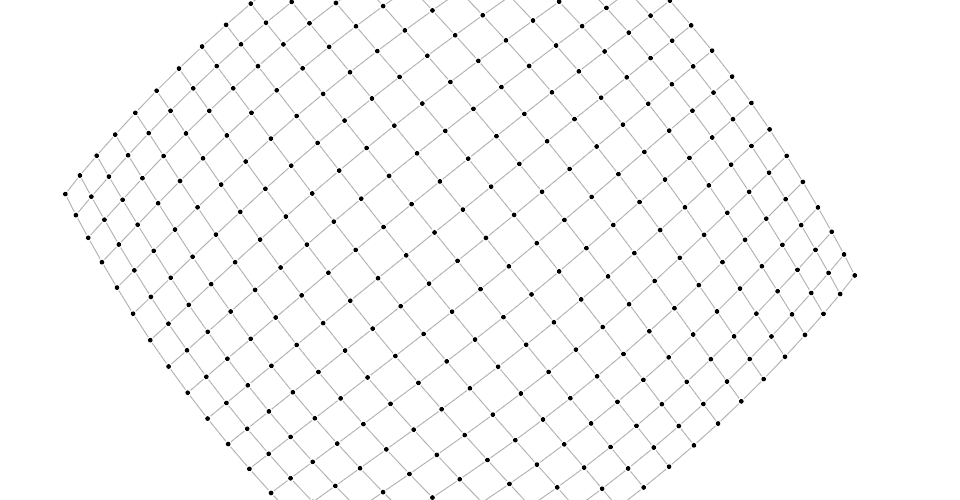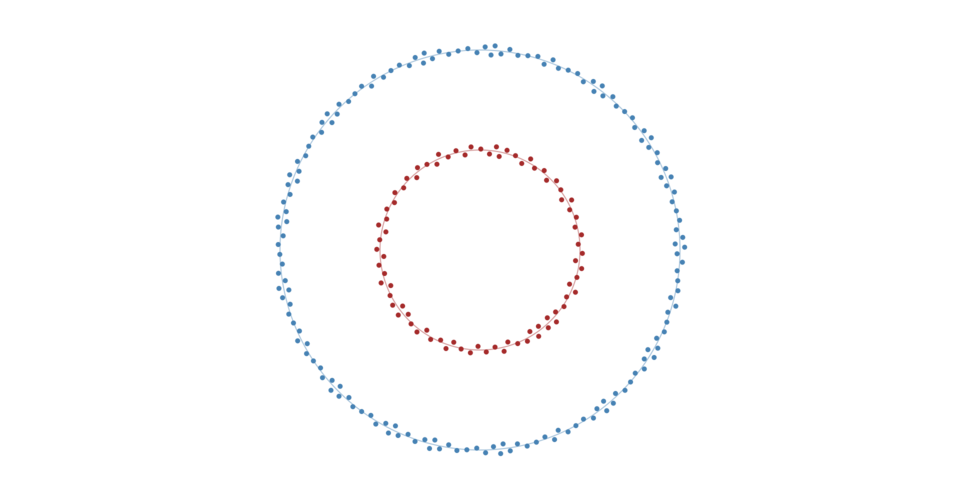# # d3-force(opens new window)

## # Installing

NPM 安装: `npm install d3-force`。 也可以下载 最新发行版 (opens new window). 此外还可以直接从 d3js.org (opens new window)标准库 (opens new window) 或作为 D3 4.0 (opens new window) 的一部分直接载入. 支持 `AMD`, `CommonJS` 以及最基本的标签引入形式，如果使用标签引入则会暴露全局 `d3` 变量:

``````<script src="https://d3js.org/d3-collection.v1.min.js"></script>
<script src="https://d3js.org/d3-dispatch.v1.min.js"></script>
<script src="https://d3js.org/d3-timer.v1.min.js"></script>
<script src="https://d3js.org/d3-force.v1.min.js"></script>
<script>

var simulation = d3.forceSimulation(nodes);

</script>
``````

## # API Reference

### # Simulation

# d3.forceSimulation([nodes]) <> (opens new window)

# simulation.restart() <> (opens new window)

# simulation.stop() <> (opens new window)

# simulation.tick() <> (opens new window)

# simulation.nodes([nodes]) <> (opens new window)

• `index` - 节点在 nodes 数组中的索引
• `x` - 节点当前的 x-坐标
• `y` - 节点当前的 y-坐标
• `vx` - 节点当前的 x-方向速度
• `vy` - 节点当前的 y-方向速度

• `fx` - 节点的固定 x-位置
• `fy` - 节点的固定 y-位置

# simulation.alpha([alpha]) <> (opens new window)

# simulation.alphaMin([min]) <> (opens new window)

# simulation.alphaDecay([decay]) <> (opens new window)

alpha 衰减系数定义了当前的 alpha 值向 target alpha 迭代的快慢。默认的目标 alpha 为 0 因此从布局形式上可以认为衰减系数决定了布局冷却的快慢。衰减系数越大，布局冷却的越快，但是衰减系数大的话会引起迭代次数不够充分，导致效果不够好。衰减系数越小，迭代次数越多，最终的布局效果越好。如果想要布局永远停不下来则可以将衰减系数设置为 0；也可以设置 target alpha 大于 minimum alpha 达到相同的效果。

# simulation.alphaTarget([target]) <> (opens new window)

# simulation.velocityDecay([decay]) <> (opens new window)

# simulation.force(name[, force]) <> (opens new window)

``````var simulation = d3.forceSimulation(nodes)
.force("charge", d3.forceManyBody())
.force("center", d3.forceCenter());
``````

``````simulation.force("charge", null);
``````

# simulation.find(x, y[, radius]) <> (opens new window)

# simulation.on(typenames, [listener]) <> (opens new window)

typenames 可以由多个由空格隔开的 typename。每个 typename 都由 type 和可选的 name 组成，用 (`.`) 连接。比如 `tick.foo``tick.bar`。也就是可以为同一种事件类型注册多个事件监听器。其中 type 必须为以下几种:

• `tick` - 仿真内部定时器每次 `tick` 之后。
• `end` - 当 alpha < alphaMin 时仿真内部定时器停止。

### # Forces

force（力模型） 是一个用以修改节点位置和速度的函数；在力模型上下文中，force 可以施加电荷或重力之类的经典物理力学，也可以用来解决几何约束，例如将节点保持在边界框内或者保持节点之间的相对距离。例如将节点移动到原点 ⟨0,0⟩ 的简单力学模型可以实现如下:

``````function force(alpha) {
for (var i = 0, n = nodes.length, node, k = alpha * 0.1; i < n; ++i) {
node = nodes[i];
node.vx -= node.x * k;
node.vy -= node.y * k;
}
}

// 只在初始化时被调用，可选的
force.initialize = function (nodes) {
// do something
}

const simulation = d3.forceSimulation()
.force('myForce', force)
``````

# force(alpha) <> (opens new window)

# force.initialize(nodes) <> (opens new window)

nodes 数组分配给此力模型。这个方法在将力模型通过 simulation.force 添加到仿真中并且通过 simulation.nodes 指定节点数组时被调用。可以在初始化阶段执行必要的工作，比如评估每个节点的参数特征，要避免在每次使用力模型时执行重复的工作。

#### # Centering

`center` (向心力) 可以将所有的节点的中心统一整体的向指定的位置 ⟨x,y⟩ 移动。这种力强制修改每个节点的位置，但是不会修改速度，因为修改速度会造成节点在期望的位置附近抖动。这种力可以辅助保持所有的节点在视口中心，与 positioning force 不同的是它不会修改节点之间的相对位置。

# d3.forceCenter([x, y]) <> (opens new window)

# center.x([x]) <> (opens new window)

# center.y([y]) <> (opens new window)

#### # Collision

`collision（碰撞力）` 将节点视为具有一定 radius 的圆，而不是点，并且阻止节点之间的重叠。从形式上来说，假设节点 a 和节点 b 是两个独立的节点，则 ab 之间最小距离为 radius(a) + radius(b)。为减少抖动，默认情况下，碰撞检测是一个可配置 strength(强度)iteration count(迭代次数) 的软约束。

# d3.forceCollide([radius]) <> (opens new window)

``````function radius() {
return 1;
}
``````

# collide.strength([strength]) <> (opens new window)

# collide.iterations([iterations]) <> (opens new window)

`link froce(弹簧模型)` 可以根据 link distance 将有关联的两个节点拉近或者推远。力的强度与被链接两个节点的距离成比例，类似弹簧力。

• `source` - 边的源节点; 参考 simulation.nodes
• `target` - 边的目标节点; 参考 simulation.nodes
• `index` - 基于 0 的在 links 中的索引, 会自动分配

# link.id([id]) <> (opens new window)

``````function id(d) {
return d.index;
}
``````

``````var nodes = [
{"id": "Alice"},
{"id": "Bob"},
{"id": "Carol"}
];

{"source": 0, "target": 1}, // Alice → Bob
{"source": 1, "target": 2} // Bob → Carol
];
``````

``````function id(d) {
return d.id;
}
``````

``````var nodes = [
{"id": "Alice"},
{"id": "Bob"},
{"id": "Carol"}
];

{"source": "Alice", "target": "Bob"},
{"source": "Bob", "target": "Carol"}
];
``````

`id` 访问器会在力模型初始化时为每个节点调用，与修改 nodeslinks 一样，并传递当前节点以及基于 0 的索引。

# link.distance([distance]) <> (opens new window)

``````function distance() {
return 30;
}
``````

# link.strength([strength]) <> (opens new window)

``````function strength(link) {
}
``````

# link.iterations([iterations]) <> (opens new window)

#### # Many-Body

`many-body`(N-body问题，译为电荷力比较容易理解) 在所有的节点之间相互作用。如果 strength 为正可以被用来模拟重力(吸引力)，如果强度为负可以用来模拟排斥力。这个力模型的实现采用四叉树以及 Barnes–Hut approximation (opens new window) 大大提高了性能。精确度可以使用 theta 参数自定义。

# d3.forceManyBody() <> (opens new window)

# manyBody.strength([strength]) <> (opens new window)

``````function strength() {
return -30;
}
``````

# manyBody.theta([theta]) <> (opens new window)

# manyBody.distanceMin([distance]) <> (opens new window)

# manyBody.distanceMax([distance]) <> (opens new window)

#### # Positioning

# d3.forceX([x]) <> (opens new window)

# x.strength([strength]) <> (opens new window)

``````function strength() {
return 0.1;
}
``````

# x.x([x]) <> (opens new window)

``````function x() {
return 0;
}
``````

x-访问器会为仿真中的每个节点进行调用，并传递当前 node 以及基于 0 的索引。计算结果会被内部存储，只有在初始化或者重新设置 x 访问器时参会重新计算，避免在每次应用时都重新计算。

# d3.forceY([y]) <> (opens new window)

# y.strength([strength]) <> (opens new window)

``````function strength() {
return 0.1;
}
``````

# y.y([y]) <> (opens new window)

``````function y() {
return 0;
}
``````

y-访问器会为仿真中的每个节点进行调用，并传递当前 node 以及基于 0 的索引。计算结果会被内部存储，只有在初始化或者重新设置 y 访问器时参会重新计算，避免在每次应用时都重新计算。(opens new window)

# radial.strength([strength]) <> (opens new window)

``````function strength() {
return 0.1;
}
``````